### Basic Integration

In this tutorial, you will learn:

• What integration is.
• Its relation to differentiation.
• Why the inverse of differentiation process becomes integration.
• How a set of special questions help you master the topic

Integration is the inverse of differentiation.In other words, if you reverse the process of differentiation, you are just doing integration. The following example shows it:
y = x2 => dy/dx = 2x
So, ∫ (dy/dx) dx = ∫ 2x dx = x2
and dx go hand in hand and indicate the integration of the function with respective to x. In the same way, s dt and indicate the integration of s with respect to dt. The outcome of the integration is called integral.

Now look at the following three examples:
y = x2 => dy/dx = 2x
y = x2 + 3 => dy/dx = 2x
y = x2 - 5 => dy/dx = 2x
So, an issue arises when it comes to integration:
We are not sure about the exact solution of ∫ 2x dx; it can be any of the above three: y = x2 or y = x2 + 3 or y = x2 - 5
In order to deal with the uncertainty, we denote the basic integration as follows: ∫ (dy/dx) dx = y + c where c is an arbitrary constant.
So, with regard to the above example,
∫ 2x dx = x2 + c, where c can be 0, 3 or -5
c shows uncertainty; it can take any value which is not certain at the time of integration. Therefore, the result is called indefinite integral.

#### Formula for integration: ∫ xn dx = xn+1/n+1 + c

E.g.1

∫x dx = x1+1/1+1 + c
= x2/2 + c

E.g.2

∫x2 dx = x2+1/2+1 + c
= x3/3 + c

E.g.3

∫a dx = ∫a (1) dx
= a ∫ x0 dx
= a x0+1/0+1 + c
= ax + c

E.g.4

∫ x1/2 dx
= x(1/2 + 1)/(1/2 + 1) + c
= x3/2/3/2 + c
= 2x3/2/3 + c

E.g.5

∫(x + 2)2 dx
∫ (x2 + 4x + 4) dx
= x3/3 + 4x2/2 + 4x + c
= x3/3 + 2x2 + 4x + c

E.g.6

∫ (x + 2)/√x dx
∫ (x/√x + 2/√x) dx
∫ (x1/2 + 2x-1/2 dx
= x3/2/3/2 + 2x1/2/1/2 + c
= 2x3/2/3 + 4x1/2 + c

#### Definite Integral

The general integration gives us a constant to signify the uncertainty of the numerical value that could be added or taken away from the result. In definite integral, there is no room for the constant, as the integration is performed between a certain range of the variable.

ab f'(x) dx = [f(x) + c ]ab
= (f(b) + c) - (f(a) + c)
= f(b) - f(a)
The constant disappears; this is the definite integral.

E.g.1

24 3x2 dx
= [3x3/3]24
= [x3]24
= 43 - 23
= 64 - 8
= 56

E.g.2

02 (x + 1)2 dx
02 (x2 + 2x + 1) dx
= [x3/3 + 2x2/2 + x]02
= [23/3 + 22 + 2] - [03/3 + 02 + 0]
= [8/3 + 4 + 2] - 
= 8.6

#### Finding the area under a curve

The area between a curve and the x-axis is the definite integral of the function of the curve within the given range of x.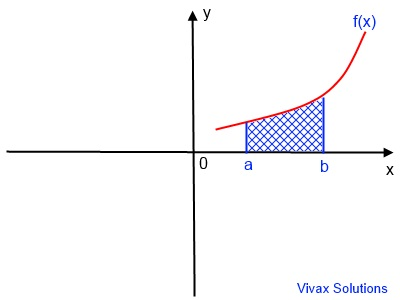#### Area = a ∫ b f(x) dx

E.g.1

Find the under the curve, f(x) = x2, for -1 <= x <= 2.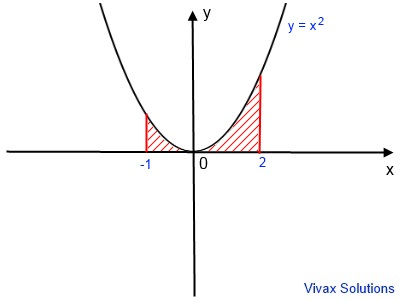Area = -12 x2 dx
= [x3/3]-12
= [23/3] - [-13/3]
= 8/3 - -1/3
= 3

E.g.2

Find the under the curve, f(x) = x(x - 2)(x + 2), for -1 <= x <= 1.Area = -11 x(x - 2)(x + 2) dx
Area = -11 x3 - 4x dx
= [x4/4 - 4x2/2]-11
= [x4/4 - 2x2]-11
= [14/4 - (2)12 - (-1)4/4 - (2)(-1)2 ]
= 0
The answer is definitely not right, because there is clearly an area between the curve and the x-axis.
To avoid the error, we have to integrate it in two parts: from x = -1 to x = 0 and x = 0 to x = 1.

Area of the left part = -10 x(x - 2)(x + 2) dx
Area of the left part= -10 x3 - 4x dx
= [x4/4 - 4x2/2]-10
= [x4/4 - 2x2]-10
= [04/4 - (2)02 - (-1)4/4 - (2)(-1)2 ]
= 7/4
= 1.75
Area of the right part = 01 x(x - 2)(x + 2) dx
Area of the right part = 01 x3 - 4x dx
= [x4/4 - 4x2/2]01
= [x4/4 - 2x2]01
= [14/4 - (2)12 - (0)4/4 - (2)(0)2 ]
= -7/4
= -1.75
Since area cannot be negative, the real value is 1.75.
So, the total area under the curve = 2 X 1.75 = 3.5

#### Area Under a Curve - Interactive

In the following applet, the area under the curve, y = x2 - 2x + 1, is calculated for the region covered by a ≤ x ≤ b. You can change the position of the sliders to change a and b to see it.

#### Area between a Line and a Curve - interactive

E.g

Find the area of the blue region, between the curve, y = x(x - 2) and the line, y = x.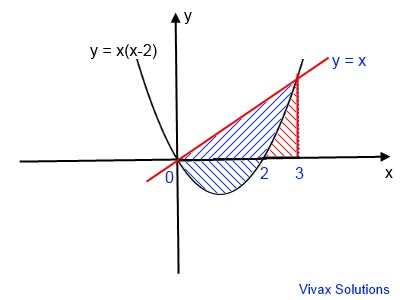First of all, let's find the point of intersection of the curve and the line.
At the point of intersection,
x(x-2) = x
x2 - 2x -x = 0
x2 - 3x = 0
x(x - 3) = 0
x = 0 or x = 3
Area below the x-axis - blue region = 02 x2 - 2x dx
= [x3/3 - x2]02
= 4/3

Area under the line between x = 0 and x = 3 = 03 x dx
= [x2/2]03
= 9/2
Area under the curve between x = 2 and x = 3 = 23 ∫ x2 - 2x dx
= [x3/3 - x2]23
= 4/3
So, the area of the blue shaded region = 9/2 - 4/3 + 4/3 = 4.5

In the following applet, the area between the line and the curve can be calculated for, 0 ≤ x ≤ 2, at which the two intersect.

#### Area of a Rectangle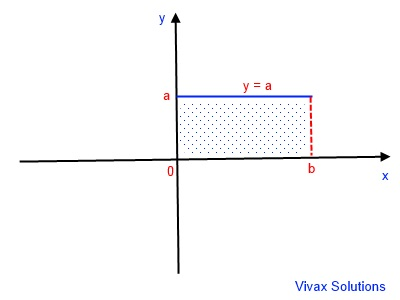The equation of the line is y = a
So, the area under the line that forms the rectangle = 0b ∫ a dx
Area = [ax]0b
Area = ab Area = length X width

#### Area of a Triangle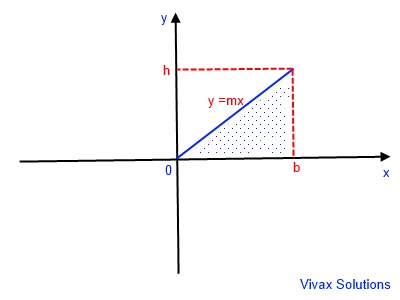The equation of the line is y = mx, where m is the gradient.
So, the area under the line that forms the triangle = 0b ∫ mx dx
Area = [mx2/2]0b
Area = mb2/2 - m0/2
Area = mb2/2
Since m, the gradient, = h/b
Area = h/b * b2/2
Area = 1/2 h * b
Area = 1/2 * height * base

Ad:The author's fully interactive tutorial on differentiation

#### Area of a Trapezoid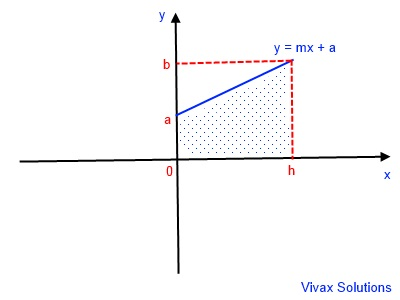The equation of the line is y = mx + a where m is the gradient and a is the y-intercept.
Area under the line that forms the trapezoid = 0h ∫mx + a dx
= [mx2/2 + ax]0h
= mh2/2 + ah
= (mh2 + 2ah)/2
= h/2 [mh + 2a]
= h/2 [(b-a)/h * h + 2a]
= h/2 [b - a + 2a]
= h/2 [b + a]
Area = height/2 [sum of the two parallel sides]

Now that you have read this tutorial, you will find the following tutorials very helpful too:Maths is challenging; so is finding the right book. K A Stroud, in this book, cleverly managed to make all the major topics crystal clear with plenty of examples; popularity of the book speak for itself - 7th edition in print.

### Recommended - GCSE & iGCSEThis is the best book available for the new GCSE(9-1) specification and iGCSE: there are plenty of worked examples; a really good collection of problems for practising; every single topic is adequately covered; the topics are organized in a logical order.

### Recommended for A LevelThis is the best book that can be recommended for the new A Level - Edexcel board: it covers every single topic in detail;lots of worked examples; ample problems for practising; beautifully and clearly presented.